# AP Chemistry : Reaction Mechanisms

## Example Questions

### Example Question #1 : Reaction Mechanisms

Which of the following is true?

Since intermediate compounds can be formed, the chemical equations for the elementary reaction in a multistep mechanism do not always have to add to give the chemical equation of the overall process.

If we know that a reaction is an elementary reaction, then we know its rate law.

The rate-determining step of a reaction is the rate of the slowest elementary step of its mechanism

All of the above

In a reaction mechanism an intermediate is identical to an activated complex

All of the above

Explanation:

All of the above describe elementary reactions and how they give an overall mechanism.

### Example Question #1 : Reaction Mechanisms

A possible mechanism for the overall reaction Br2 (g) + 2 NO (g)  ->  2 NOBr(g) is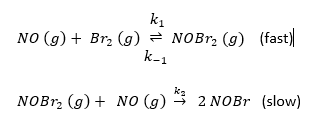The rate law for the formation of NOBr based on this mechanism is rate =           .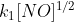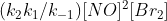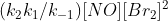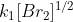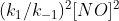Explanation:

Based on the slowest step the rate law would be:  Rate = k2 [NOBr2] [NO], but one cannot have a rate law in terms of an intermediate (NOBr2).

Because the first reaction is at equilibrium the rate in the forward direction is equal to that in the reverse, thus: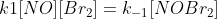and: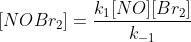Substitution yields: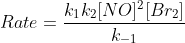### Example Question #1 : Reaction Mechanisms

For the reaction NO2 (g) + CO (g) -> NO (g) + CO2 (g), the reaction was experimentally determined to be Rate = k[NO2]2.  If the reaction has the following mechanism, what is the rate limiting step, and why?

Step 1:  2 NO2  -> NO3 + NO                            (slow)

Step 2:  NO3 + CO -> NO2 + CO2                     (fast)

Not enough information

Step 2 is limiting because the fast step determines how quickly the reaction can occur.

Step 1 is limiting because the NO2 is a reactant.

Step 1 is limiting because the reaction can not go faster than its slowest step.

Step 2 is limiting because the NO3 intermediate has to be formed before the reaction can occur.

Step 1 is limiting because the reaction can not go faster than its slowest step.

Explanation:

The reaction can never go faster than its slowest step.

### Example Question #4 : Reaction Mechanisms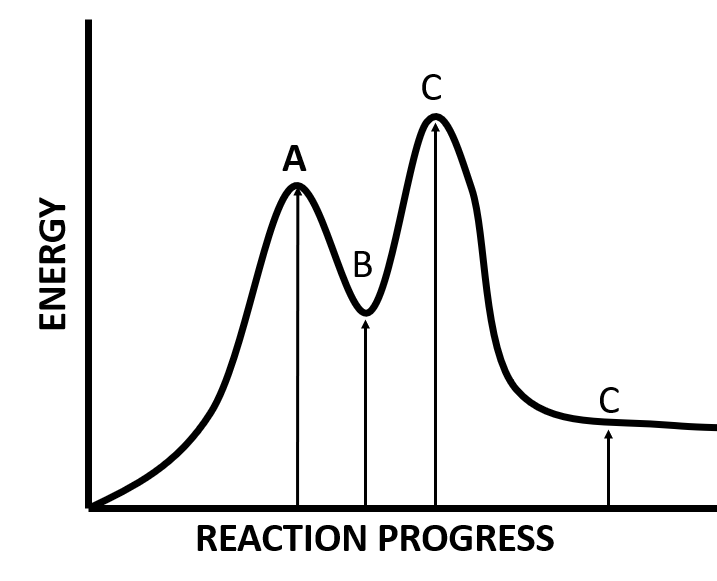Based on the figure above, what arrows corresponds to the activation energy of the rate limiting step and the energy of reaction?  Is the reaction endo- or exothermic?

Exothermic

Endothermic

Endothermic

Explanation:

Since the products are higher in energy than the reactions, the reaction is endothermic.

### Example Question #1 : Reaction Mechanisms

Consider the following mechanism:

A + B ->  R +  C              (slow)

A + R  ->  C                   (fast)

There are no intermediates

R

C

B

A